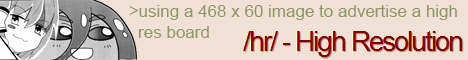[a / b / c / d / e / f / g / gif / h / hr / k / m / o / p / r / s / t / u / v / vg / vm / vmg / vr / vrpg / vst / w / wg] [i / ic] [r9k / s4s / vip / qa] [cm / hm / lgbt / y] [3 / aco / adv / an / bant / biz / cgl / ck / co / diy / fa / fit / gd / hc / his / int / jp / lit / mlp / mu / n / news / out / po / pol / pw / qst / sci / soc / sp / tg / toy / trv / tv / vp / vt / wsg / wsr / x / xs] [Settings] [Search] [Mobile] [Home]
Board
/sci/ - Science & MathI broke Wolfram Alpha
>>
>WA doesn't use infinite bits
well duh
>>
Ha, ha. Now it's like that dumb kid in a math exam that accidentally gets the right answer. It actually doesn't know that this expression has a division by zero. Even if you input just the denominator, it still says it's undefined even though it should be zero in that case.
>>
Now it's giving more creative answers
>>
thats ok, I broke math

>exponents are not equal
>power rule is false

999... / 1000... = 0.999... = 3 * 1/3 = 9/9 = 1
>these are apparently all equivilant
>>
>>14596240
fake news, I just tried wolfram alpha and it still works. not broken.
>>
>>14596240
>>14596273
>Let me just allocate petabits of numbers specially for you to calculate your autistic equation
>REEEEE you're broken!
meds
>>
>>14597217
You didn't break anything...it's literally giving the correct answer. Square root of x^2 is abs(x) so x does not equal +/x...right side is always positive. Next line is assuming x is positive so it is true.

You're the dumbest fuck on this board today, and that's a huge accomplishment.
>>
>>14597731
You sound mad I broke you religion
Tell me again how the power rule holds true with half of all numbers?
Or are you saying that 'i' = +/-1?
>>
>>14598266
And yes...sqrt(x^2) is +/-x ... literally high school math...so the expressions you wrote are only equal when x>0 which is what the 2nd line is clarifying.
>>
>>14598288
>>
>>14598266
sqrt(x^2) =/= sqrt(x)^2 ... it's the way you wrote the parenthesis
>>
>>14598303
That is what I wrote yes, but power law reduction would say x = x when it does not, therefore power law is incorrect, therefore all exponential reduction and simplification is incorrect on half the number line.
>>
>>14598323
https://en.wikipedia.org/wiki/Exponentiation#Identities_and_properties
>The following identities, often called exponent rules, hold for all (((integer exponents))), provided that the base is non-zero:
>>
>>14597456
It should try to simplify it
>>
is everyone ITT 15 years old?

genuine question
>>
>>14596273
You could have easily programmed it to solve this based on the math formulas relating to exponentiation. The human brain doesn't use infinite information either and yet people can solve it. Furthermore it could have been made to give an error message or something instead of misleadingly saying undefined as if the expression was actually undefined.
>>
>>14598341
-1 is non zero
>>
>>14598341
>in b4 integer exponents
https://en.wikipedia.org/wiki/Exponentiation#Failure_of_power_and_logarithm_identities

Delete Post: [File Only] Style: